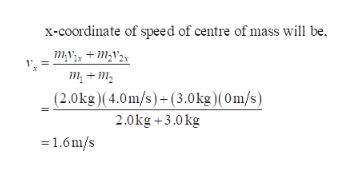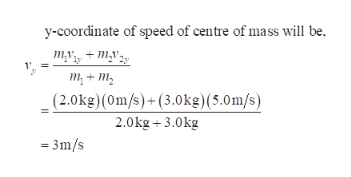# At the instant a 2.0-kg particle has a velocity of 4.0 m/s in thepositive x direction, a 3.0-kg particle has a velocity of 5.0 m/s in thepositive y direction. What is the speed of the center of mass of thetwo-particle system?

Question
32 views

At the instant a 2.0-kg particle has a velocity of 4.0 m/s in the
positive x direction, a 3.0-kg particle has a velocity of 5.0 m/s in the
positive y direction. What is the speed of the center of mass of the
two-particle system?

check_circle

Step 1

x-coordinate of speed of centre of mass will be,help_outlineImage Transcriptionclosex-coordinate of speed of centre of mass will be, m,V3, + M;V25 1. = т, + т, (2.0kg)(4.0m/s)+(3.0kg)(0m/s) 2.0kg +3.0 kg = 1.6m/s fullscreen
Step 2help_outlineImage Transcriptionclosey-coordinate of speed of centre of mass will be, myy, + m,v 35 m, + m, (2.0kg)(0m/s)+(3.0kg)(5.0m/s) 2.0kg + 3.0kg = 3m/s fullscreen
Step 3

### Want to see the full answer?

See Solution

#### Want to see this answer and more?

Solutions are written by subject experts who are available 24/7. Questions are typically answered within 1 hour.*

See Solution
*Response times may vary by subject and question.
Tagged in

### Centre of Mass and Centre of Gravity